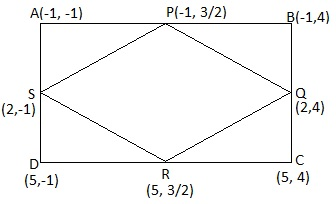Newbie

# ABCD is a rectangle formed by the points A (-1, – 1), B (-1, 4), C (5, 4) and D (5, -1). P, Q, R and S are the midpoints of AB, BC, CD and DA respectively. Is the quadrilateral PQRS a square? a rectangle? or a rhombus? Justify your answer. Q.8

• 0

How i can solve the problem of ncert class 10 coordinate geometry question easily . What is the best way to solve the exercise 7.4 question no.8 easily  , please give me easy  solution  for this question. ABCD is a rectangle formed by the points A (-1, – 1), B (-1, 4), C (5, 4) and D (5, -1). P, Q, R and S are the midpoints of AB, BC, CD and DA respectively. Is the quadrilateral PQRS a square? a rectangle? or a rhombus? Justify your answer.

Share

1.P id the mid-point of side AB,

Coordinate of P = ( (-1 – 1)/2, (-1 + 4)/2 ) = (-1, 3/2)

Similarly, Q, R and S are (As Q is mid-point of BC, R is midpoint of CD and S is midpoint of AD)

Coordinate of Q = (2, 4)

Coordinate of R = (5, 3/2)

Coordinate of S = (2, -1)

Now,

Length of PQ = √[(-1 – 2)2 + (3/2 – 4)2] = √(61/4) = √61/2

Length of SP = √[(2 + 1)2 + (-1 – 3/2)2] = √(61/4) = √61/2

Length of QR = √[(2 – 5)2 + (4 – 3/2)2] = √(61/4) = √61/2

Length of RS = √[(5 – 2)2 + (3/2 + 1)2] = √(61/4) = √61/2

Length of PR (diagonal) = √[(-1 – 5)2 + (3/2 – 3/2)2] = 6

Length of QS (diagonal) = √[(2 – 2)2 + (4 + 1)2] = 5

The above values show that, PQ = SP = QR = RS = √61/2, i.e. all sides are equal.

But PR ≠ QS i.e. diagonals are not of equal measure.

Hence, the given figure is a rhombus

• 0